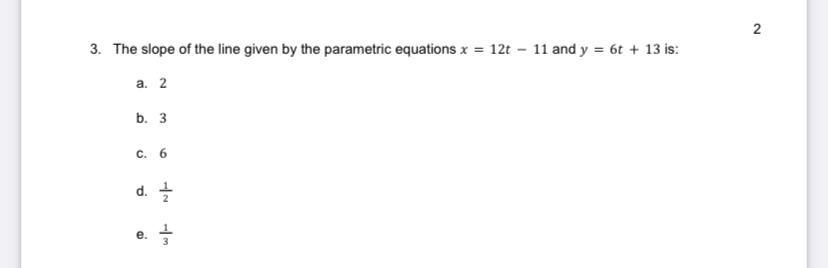Question:

# The slope of the line given by the parametric equations x

Last updated: 8/11/2022The slope of the line given by the parametric equations x =12t-11 and y = 6t+13 is: a. 2 b.3 c. 6 d. 1/2 e.1/3